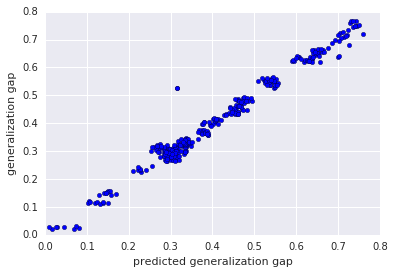# Predicting the Generalization Gap in Deep Neural Networks

Deep neural networks (DNN) are the cornerstone of recent progress in machine learning, and are responsible for recent breakthroughs in a variety of tasks such as image recognition, image segmentation, machine translation and more. However, despite their ubiquity, researchers are still attempting to fully understand the underlying principles that govern them. In particular, classical theories (e.g., VC-dimension and Rademacher complexity) suggest that over-parameterized functions should generalize poorly to unseen data, yet recent work has found that massively over-parameterized functions (orders of magnitude more parameters than the number of data points) generalize well. In order to improve models, a better understanding of generalization, which can lead to more theoretically grounded and therefore more principled approaches to DNN design, is required.

An important concept for understanding generalization is the generalization gap, i.e., the difference between a model’s performance on training data and its performance on unseen data drawn from the same distribution. Significant strides have been made towards deriving better DNN generalization bounds—the upper limit to the generalization gap—but they still tend to greatly overestimate the actual generalization gap, rendering them uninformative as to why some models generalize so well. On the other hand, the notion of margin—the distance between a data point and the decision boundary—has been extensively studied in the context of shallow models such as support-vector machines, and is found to be closely related to how well these models generalize to unseen data. Because of this, the use of margin to study generalization performance has been extended to DNNs, resulting in highly refined theoretical upper bounds on the generalization gap, but has not significantly improved the ability to predict how well a model generalizes.An example of a support-vector machine decision boundary. The hyperplane defined by w∙x-b=0 is the "decision boundary" of this linear classifier, i.e., every point x lying on the hyperplane is equally likely to be in either class under this classifier.
In our ICLR 2019 paper, “Predicting the Generalization Gap in Deep Networks with Margin Distributions”, we propose the use of a normalized margin distribution across network layers as a predictor of the generalization gap. We empirically study the relationship between the margin distribution and generalization and show that, after proper normalization of the distances, some basic statistics of the margin distributions can accurately predict the generalization gap. We also make available all the models used as a dataset for studying generalization through the Github repository.Each plot corresponds to a convolutional neural network trained on CIFAR-10 with different classification accuracies. The probability density (y-axis) of normalized margin distributions (x-axis) at 4 layers of a network is shown for three different models with increasingly better generalization (left to right). The normalized margin distributions are strongly correlated with test accuracy, which suggests they can be used as a proxy for predicting a network's generalization gap. Please see our paper for more details on these networks.
Margin Distributions as a Predictor of Generalization
Intuitively, if the statistics of the margin distribution are truly predictive of the generalization performance, a simple prediction scheme should be able to establish the relationship. As such, we chose linear regression to be the predictor. We found that the relationship between the generalization gap and the log-transformed statistics of the margin distributions is almost perfectly linear (see figure below). In fact, the proposed scheme produces better prediction relative to other existing measures of generalization. This indicates that the margin distributions may contain important information about how deep models generalize.Predicted generalization gap (x-axis) vs. true generalization gap (y-axis) on CIFAR-100 + ResNet-32. The points lie close to the diagonal line, which indicates that the predicted values of the log linear model fit the true generalization gap very well.
The Deep Model Generalization Dataset
In addition to our paper, we are introducing the Deep Model Generalization (DEMOGEN) dataset, which consists of of 756 trained deep models, along with their training and test performance on the CIFAR-10 and CIFAR-100 datasets. The models are variants of CNNs (with architectures that resemble Network-in-Network) and ResNet-32 with different popular regularization techniques and hyperparameter settings, inducing a wide spectrum of generalization behaviors. For example, the models of CNNs trained on CIFAR-10 have the test accuracies ranging from 60% to 90.5% with generalization gaps ranging from 1% to 35%. For details of the dataset, please see our paper or the Github repository. As part of the dataset release, we also include utilities to easily load the models and reproduce the results presented in our paper.

We hope that this research and the DEMOGEN dataset will provide the community with an accessible tool for studying generalization in deep learning without having to retrain a large number of models. We also hope that our findings will motivate further research in generalization gap predictors and margin distributions in the hidden layers.